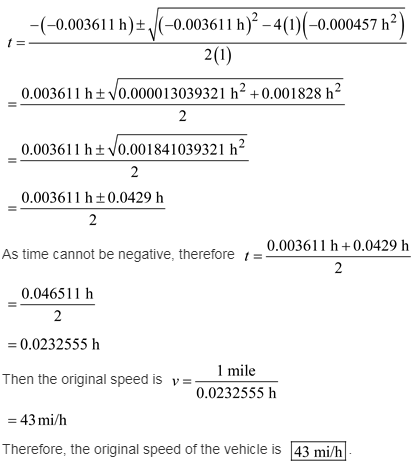# Driving along a crowded freeway, you notice that it takes a time t to go from one mile marker to the next

Driving along a crowded freeway, you notice that it takes a time t to go from one mile marker to the next. When you increase your speed by 7.9 mi/h, the time to go one mile decreases by 13 s. What was your original speed?

Use the relation between speed, distance travelled, and time of travel in two cases to solve for the original speed of the vehicle.
The expression for the average speed of the vehicle is,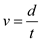Here, d is the distance traveled and t is the time of travel.
Substitute 1 mile for d.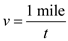Now the speed is increased by 7.9mi/h,
Therefore, the expression for new speed is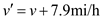New time of travel is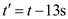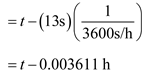The expression for new average speed is,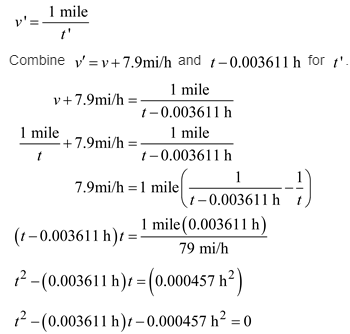The above equation is a quadratic equation in t.
Solving for t yields,